## 1 多目标跟踪中的相机运动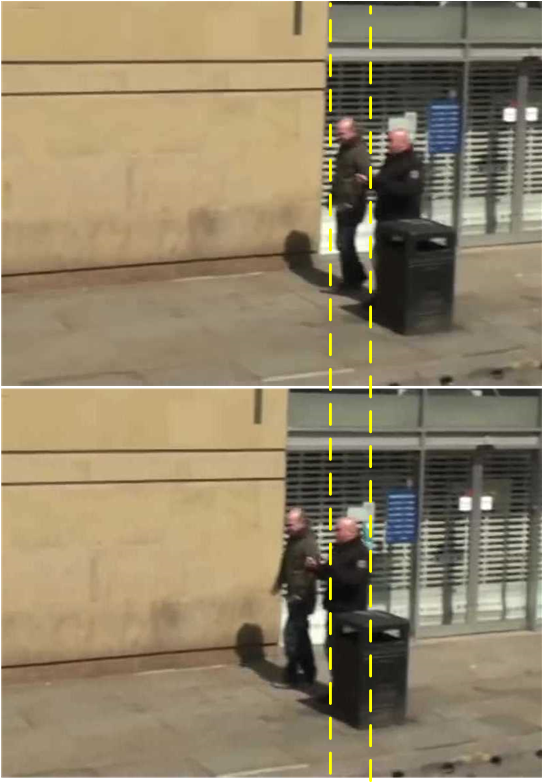## 2对极几何

### 2.1 对极几何模型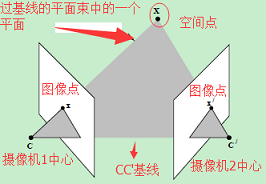1. 基线[baseline]：直线CC’为基线。
2. 对极平面束[epipolar pencil]：以基线为轴的平面束。
3. 对极平面[epipolar plane]：任何包含基线的平面都称为对极平面。
4. 对极点[epipole]：摄像机的基线与每幅图像的交点。比如，上图中的点x和x’。
5. 对极线[epipolar line]：对极平面与图像的交线。
6. 5点共面：点x，x’，摄像机中心C、C’，空间点X是5点共面的。
7. 极线约束：两极线上点的对应关系。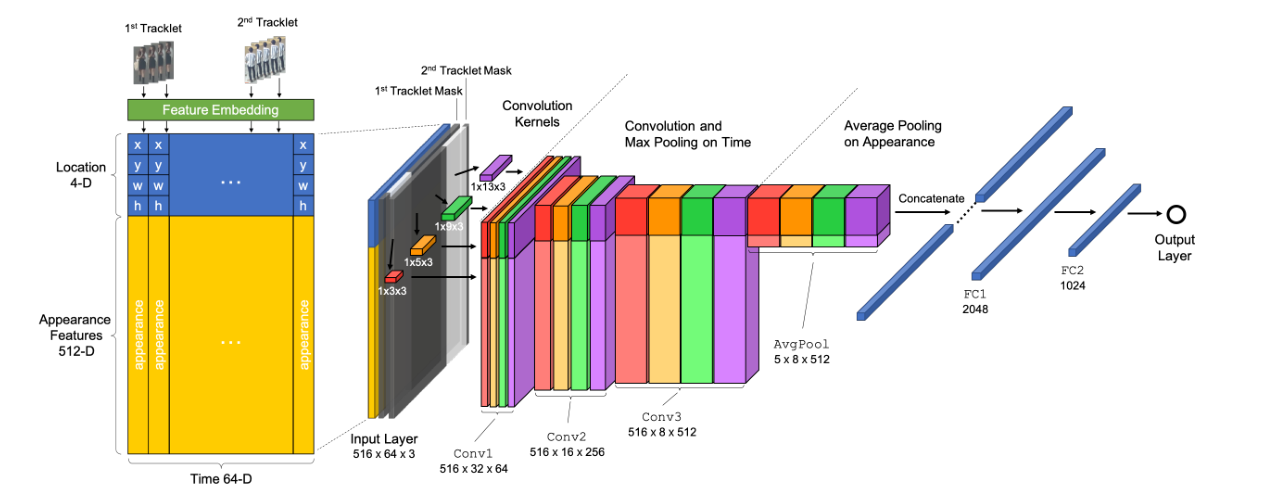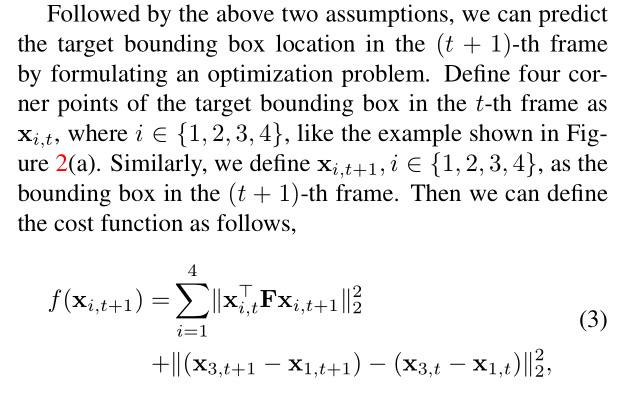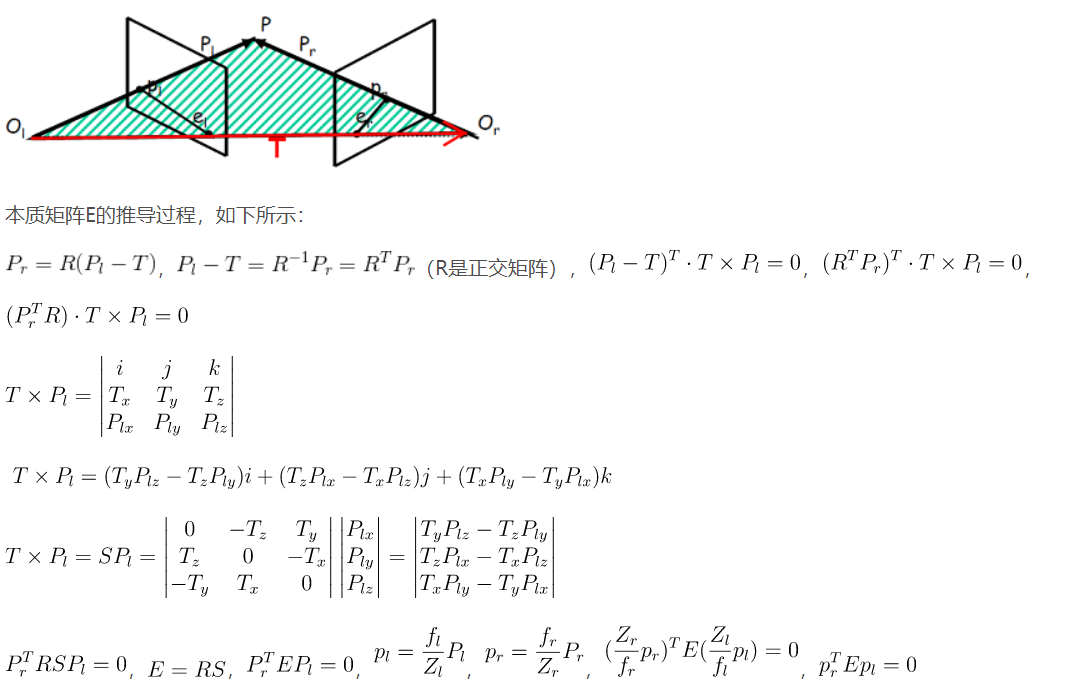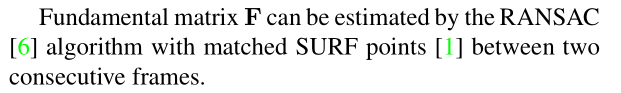$$\begin{array}{l} \left\{ \begin{array}{l} {M_{11}}x + {M_{12}}y + {M_{13}} = 0\\ {M_{21}}\left( {x + w} \right) + {M_{22}}y + {M_{23}} = 0\\ {M_{31}}x + {M_{32}}\left( {y + h} \right) + {M_{33}} = 0\\ {M_{41}}\left( {x + w} \right) + {M_{42}}\left( {y + h} \right) + {M_{43}} = 0 \end{array} \right.\\ \Rightarrow \left[ {\begin{array}{*{20}{c}} {{M_{11}}}&{{M_{12}}}\\ {{M_{21}}}&{{M_{22}}}\\ {{M_{31}}}&{{M_{32}}}\\ {{M_{41}}}&{{M_{42}}} \end{array}} \right]\left[ {\begin{array}{*{20}{c}} x\\ y \end{array}} \right] = \left[ {\begin{array}{*{20}{c}} {{M_{13}}}\\ {{M_{23}}{\rm{ + }}w{M_{21}}}\\ {{M_{33}}{\rm{ + }}h{M_{32}}}\\ {{M_{43}}{\rm{ + }}w{M_{41}} + h{M_{42}}} \end{array}} \right] \end{array}$$

### 2.2 实验分析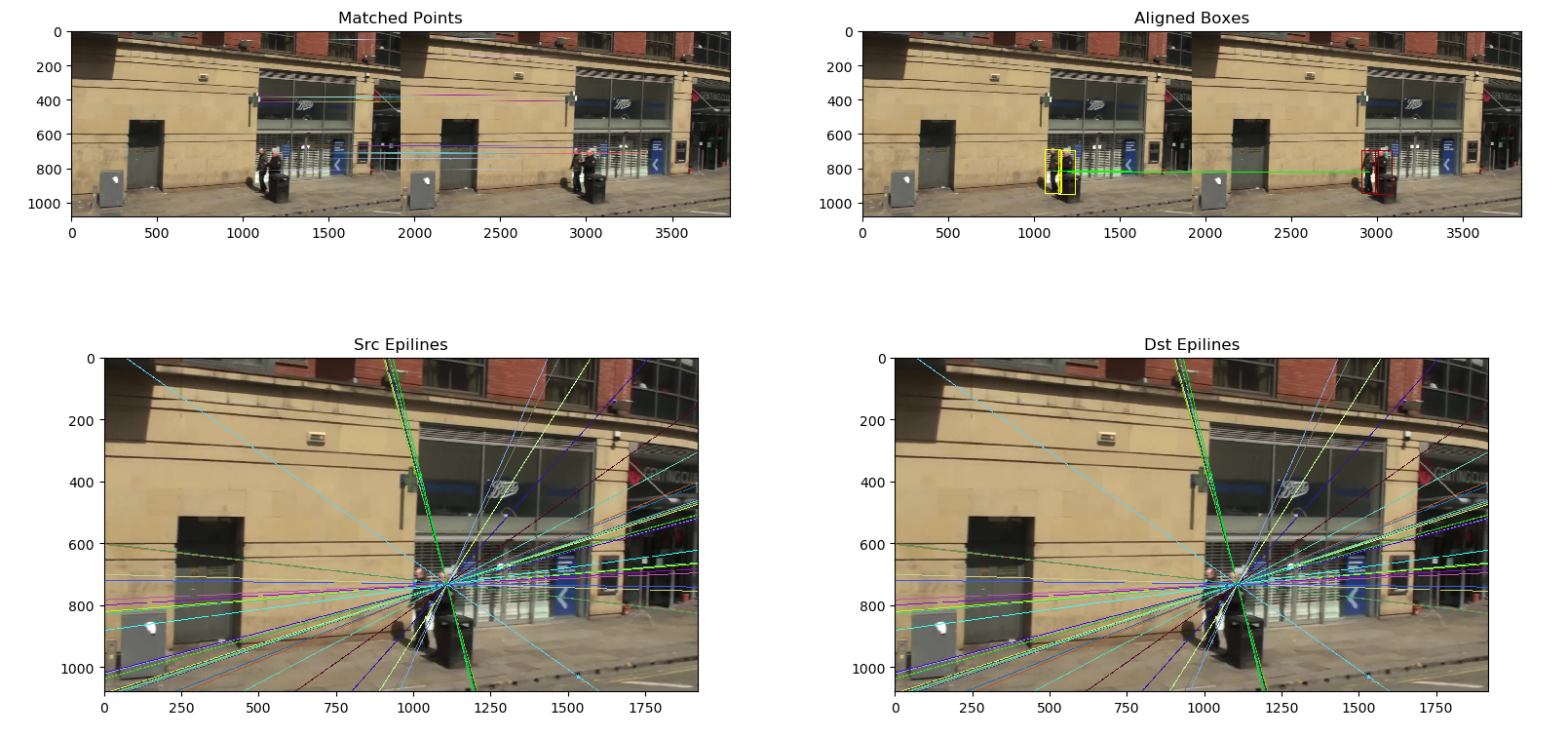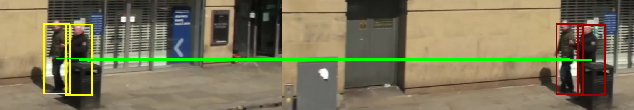## 3ECC

### 3.1原理介绍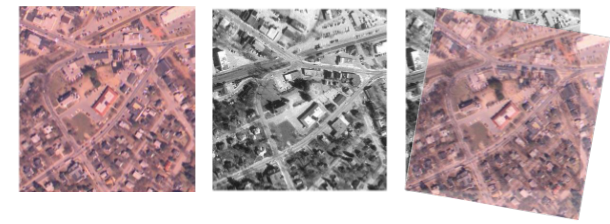$$ECC = {\left\| {\frac{{{x_i}}}{{\left\| {{x_i}} \right\|}} - \frac{{{y_i}}}{{\left\| {{y_i}} \right\|}}} \right\|^2},y = warp(x)$$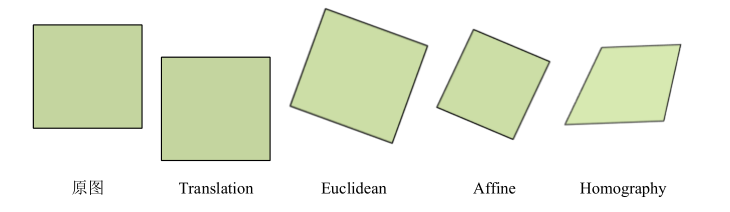$$\left[ {\begin{array}{*{20}{c}} {x'}\\ {y'}\\ {z'} \end{array}} \right] = \left[ {\begin{array}{*{20}{c}} {{a_{11}}}&{{a_{12}}}&{{t_x}}\\ {{a_{21}}}&{{a_{22}}}&{{t_y}}\\ {{a_{23}}}&{{a_{24}}}&{{t_z}} \end{array}} \right]\left[ {\begin{array}{*{20}{c}} x\\ y\\ z \end{array}} \right]$$

### 3.2实验分析

opencv中正好提供了ECC相关的功能函数，这里我们只需要再次封装，以方便多目标跟踪。可以知道的是ECC算法的核心在于变换矩阵的求解：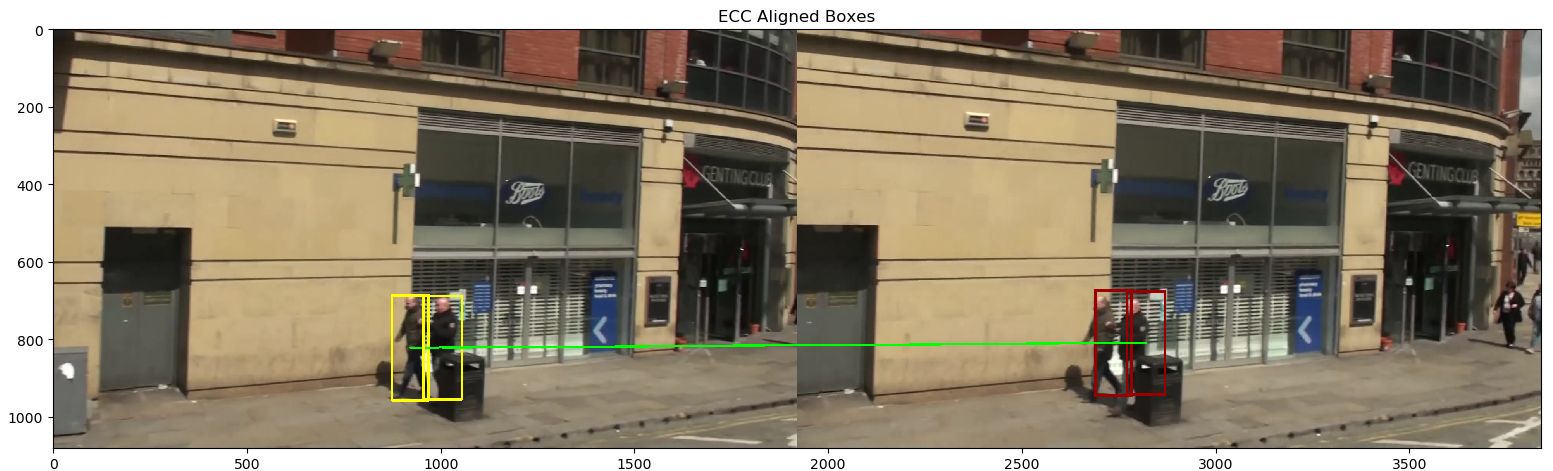## 4 其他近似方案

### 4.1光流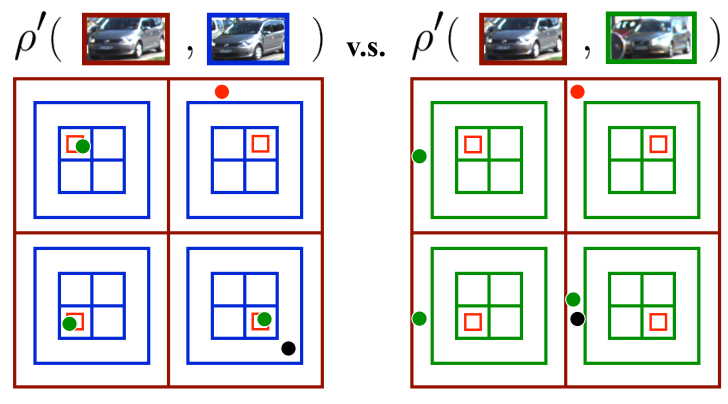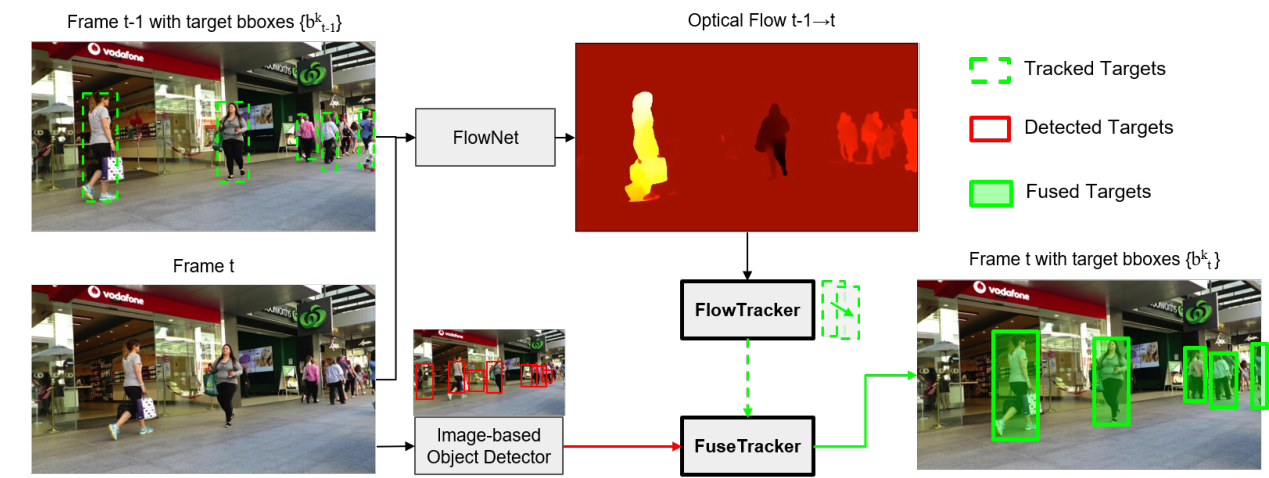### 4.2SOT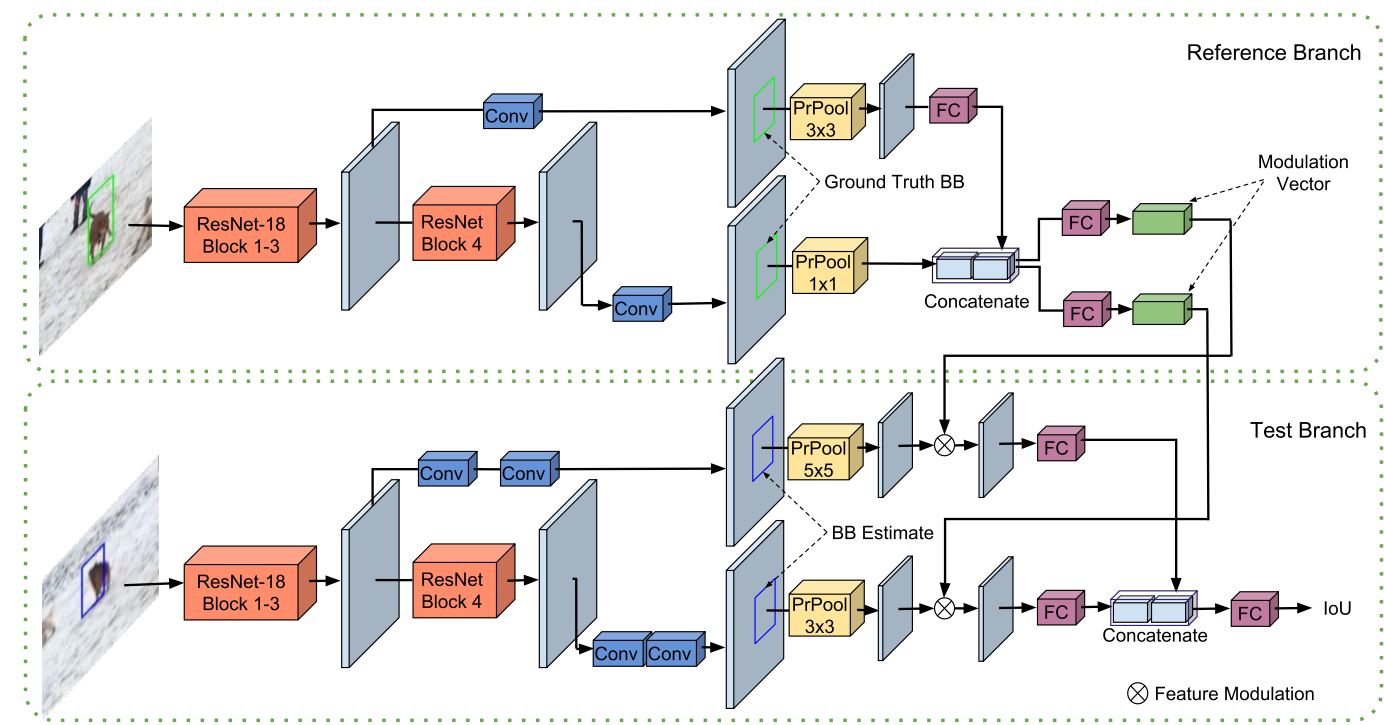## 参考资料

 Wang G, Wang Y, Zhang H, et al. Exploit the connectivity: Multi-object tracking with trackletnet[C]. in: Proceedings of the 27th ACM International Conference on Multimedia. 2019. 482-490.

 Evangelidis G D, Psarakis E Z. Parametric image alignment using enhanced correlation coefficient maximization[J]. IEEE transactions on pattern analysis and machine intelligence, 2008, 30(10): 1858-1865.

 Bergmann P, Meinhardt T, Leal-Taixe L. Tracking without bells and whistles[C]. in: Proceedings of the IEEE International Conference on Computer Vision. 2019. 941-951.

 Choi W. Near-online multi-target tracking with aggregated local flow descriptor[C]. in: Proceedings of the IEEE international conference on computer vision. 2015. 3029-3037.

 Feng W, Hu Z, Wu W, et al. Multi-object tracking with multiple cues and switcher-aware classification[J]. arXiv preprint arXiv:1901.06129, 2019.

-------------本文结束感谢您的阅读-------------